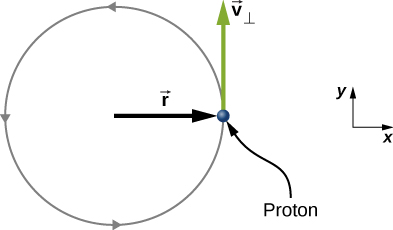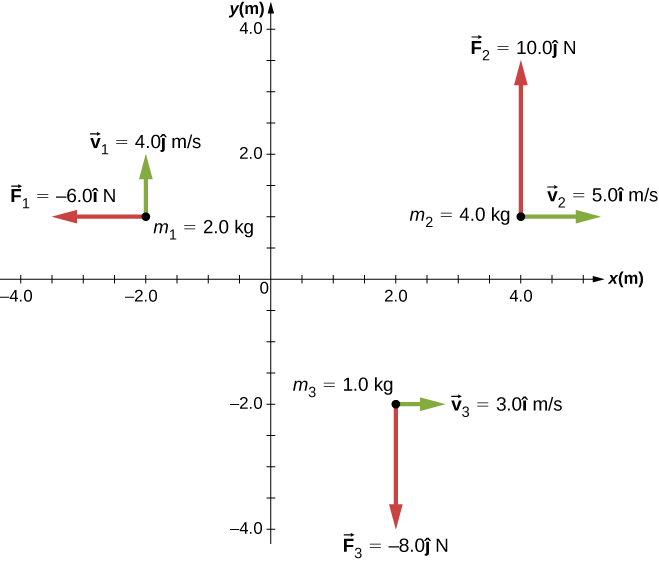# 11.2 Angular momentum  (Page 3/8)

 Page 3 / 8

## Significance

Since the meteor is accelerating downward toward Earth, its radius and velocity vector are changing. Therefore, since $\stackrel{\to }{l}=\stackrel{\to }{r}\phantom{\rule{0.2em}{0ex}}×\phantom{\rule{0.2em}{0ex}}\stackrel{\to }{p}$ , the angular momentum is changing as a function of time. The torque on the meteor about the origin, however, is constant, because the lever arm ${\stackrel{\to }{r}}_{\perp }$ and the force on the meteor are constants. This example is important in that it illustrates that the angular momentum depends on the choice of origin about which it is calculated. The methods used in this example are also important in developing angular momentum for a system of particles and for a rigid body.

Check Your Understanding A proton spiraling around a magnetic field executes circular motion in the plane of the paper, as shown below. The circular path has a radius of 0.4 m and the proton has velocity $4.0\phantom{\rule{0.2em}{0ex}}×\phantom{\rule{0.2em}{0ex}}{10}^{6}\phantom{\rule{0.2em}{0ex}}\text{m}\text{/}\text{s}$ . What is the angular momentum of the proton about the origin?From the figure, we see that the cross product of the radius vector with the momentum vector gives a vector directed out of the page. Inserting the radius and momentum into the expression for the angular momentum, we have
$\stackrel{\to }{l}=\stackrel{\to }{r}\phantom{\rule{0.2em}{0ex}}×\phantom{\rule{0.2em}{0ex}}\stackrel{\to }{p}=\left(0.4\phantom{\rule{0.2em}{0ex}}\text{m}\stackrel{^}{i}\text{)}\phantom{\rule{0.2em}{0ex}}×\phantom{\rule{0.2em}{0ex}}\left(1.67\phantom{\rule{0.2em}{0ex}}×\phantom{\rule{0.2em}{0ex}}{10}^{-27}\phantom{\rule{0.2em}{0ex}}\text{kg}\left(4.0\phantom{\rule{0.2em}{0ex}}×\phantom{\rule{0.2em}{0ex}}{10}^{6}\phantom{\rule{0.2em}{0ex}}\text{m}\text{/}\text{s}\right)\stackrel{^}{j}\right)=2.7\phantom{\rule{0.2em}{0ex}}×\phantom{\rule{0.2em}{0ex}}{10}^{-21}\phantom{\rule{0.2em}{0ex}}\text{kg}·{\text{m}}^{2}\text{/}\text{s}\stackrel{^}{k}$

## Angular momentum of a system of particles

The angular momentum of a system of particles is important in many scientific disciplines, one being astronomy. Consider a spiral galaxy, a rotating island of stars like our own Milky Way. The individual stars can be treated as point particles, each of which has its own angular momentum. The vector sum of the individual angular momenta give the total angular momentum of the galaxy. In this section, we develop the tools with which we can calculate the total angular momentum of a system of particles.

In the preceding section, we introduced the angular momentum of a single particle about a designated origin. The expression for this angular momentum is $\stackrel{\to }{l}=\stackrel{\to }{r}\phantom{\rule{0.2em}{0ex}}×\phantom{\rule{0.2em}{0ex}}\stackrel{\to }{p},$ where the vector $\stackrel{\to }{r}$ is from the origin to the particle, and $\stackrel{\to }{p}$ is the particle’s linear momentum. If we have a system of N particles, each with position vector from the origin given by ${\stackrel{\to }{r}}_{i}$ and each having momentum ${\stackrel{\to }{p}}_{i},$ then the total angular momentum of the system of particles about the origin is the vector sum of the individual angular momenta about the origin. That is,

$\stackrel{\to }{L}={\stackrel{\to }{l}}_{1}+{\stackrel{\to }{l}}_{2}+\cdots +{\stackrel{\to }{l}}_{N}.$

Similarly, if particle i is subject to a net torque ${\stackrel{\to }{\tau }}_{i}$ about the origin, then we can find the net torque about the origin due to the system of particles by differentiating [link] :

$\frac{d\stackrel{\to }{L}}{dt}=\sum _{i}\frac{d{\stackrel{\to }{l}}_{i}}{dt}=\sum _{i}{\stackrel{\to }{\tau }}_{i}.$

The sum of the individual torques produces a net external torque on the system, which we designate $\sum \stackrel{\to }{\tau }.$ Thus,

$\frac{d\stackrel{\to }{L}}{dt}=\sum \stackrel{\to }{\tau }.$

[link] states that the rate of change of the total angular momentum of a system is equal to the net external torque acting on the system when both quantities are measured with respect to a given origin. [link] can be applied to any system that has net angular momentum, including rigid bodies, as discussed in the next section.

## Angular momentum of three particles

Referring to [link] (a), determine the total angular momentum due to the three particles about the origin. (b) What is the rate of change of the angular momentum?Three particles in the xy- plane with different position and momentum vectors.

## Strategy

Write down the position and momentum vectors for the three particles. Calculate the individual angular momenta and add them as vectors to find the total angular momentum. Then do the same for the torques.

## Solution

1. Particle 1: ${\stackrel{\to }{r}}_{1}=-2.0\phantom{\rule{0.2em}{0ex}}\text{m}\stackrel{^}{i}+1.0\phantom{\rule{0.2em}{0ex}}\text{m}\stackrel{^}{j},\phantom{\rule{0.5em}{0ex}}{\stackrel{\to }{p}}_{1}=2.0\phantom{\rule{0.2em}{0ex}}\text{kg}\left(4.0\phantom{\rule{0.2em}{0ex}}\text{m}\text{/}\text{s}\stackrel{^}{j}\right)=8.0\phantom{\rule{0.2em}{0ex}}\text{kg}·\text{m}\text{/}\text{s}\stackrel{^}{j},$
${\stackrel{\to }{l}}_{1}={\stackrel{\to }{r}}_{1}\phantom{\rule{0.2em}{0ex}}×\phantom{\rule{0.2em}{0ex}}{\stackrel{\to }{p}}_{1}=-16.0\phantom{\rule{0.2em}{0ex}}\text{kg}·{\text{m}}^{2}\text{/}\text{s}\stackrel{^}{k}.$

Particle 2: ${\stackrel{\to }{r}}_{2}=4.0\phantom{\rule{0.2em}{0ex}}\text{m}\stackrel{^}{i}+1.0\phantom{\rule{0.2em}{0ex}}\text{m}\stackrel{^}{j},\phantom{\rule{0.5em}{0ex}}{\stackrel{\to }{p}}_{2}=4.0\phantom{\rule{0.2em}{0ex}}\text{kg}\left(5.0\phantom{\rule{0.2em}{0ex}}\text{m}\text{/}\text{s}\stackrel{^}{i}\right)=20.0\phantom{\rule{0.2em}{0ex}}\text{kg}·\text{m}\text{/}\text{s}\stackrel{^}{i}$ ,
${\stackrel{\to }{l}}_{2}={\stackrel{\to }{r}}_{2}\phantom{\rule{0.2em}{0ex}}×\phantom{\rule{0.2em}{0ex}}{\stackrel{\to }{p}}_{2}=-20.0\phantom{\rule{0.2em}{0ex}}\text{kg}·{\text{m}}^{2}\text{/}\text{s}\stackrel{^}{k}.$

Particle 3: ${\stackrel{\to }{r}}_{3}=2.0\phantom{\rule{0.2em}{0ex}}\text{m}\stackrel{^}{i}-2.0\phantom{\rule{0.2em}{0ex}}\text{m}\stackrel{^}{j},\phantom{\rule{0.5em}{0ex}}{\stackrel{\to }{p}}_{3}=1.0\phantom{\rule{0.2em}{0ex}}\text{kg}\left(3.0\phantom{\rule{0.2em}{0ex}}\text{m}\text{/}\text{s}\stackrel{^}{i}\right)=3.0\phantom{\rule{0.2em}{0ex}}\text{kg}·\text{m}\text{/}\text{s}\stackrel{^}{i}$ ,
${\stackrel{\to }{l}}_{3}={\stackrel{\to }{r}}_{3}\phantom{\rule{0.2em}{0ex}}×\phantom{\rule{0.2em}{0ex}}{\stackrel{\to }{p}}_{3}=6.0\phantom{\rule{0.2em}{0ex}}\text{kg}·{\text{m}}^{2}\text{/}\text{s}\stackrel{^}{k}.$

We add the individual angular momenta to find the total about the origin:
${\stackrel{\to }{l}}_{T}={\stackrel{\to }{l}}_{1}+{\stackrel{\to }{l}}_{2}+{\stackrel{\to }{l}}_{3}=-30\phantom{\rule{0.2em}{0ex}}\text{kg}·{\text{m}}^{2}\text{/}\text{s}\stackrel{^}{k}.$
2. The individual forces and lever arms are
$\begin{array}{c}{\stackrel{\to }{r}}_{1\perp }=1.0\phantom{\rule{0.2em}{0ex}}\text{m}\stackrel{^}{j},\phantom{\rule{0.5em}{0ex}}{\stackrel{\to }{F}}_{1}=-6.0\phantom{\rule{0.2em}{0ex}}\text{N}\stackrel{^}{i},\phantom{\rule{0.5em}{0ex}}{\stackrel{\to }{\tau }}_{1}=6.0\text{N}·\text{m}\stackrel{^}{k}\hfill \\ {\stackrel{\to }{r}}_{2\perp }=4.0\phantom{\rule{0.2em}{0ex}}\text{m}\stackrel{^}{i},\phantom{\rule{0.5em}{0ex}}{\stackrel{\to }{F}}_{2}=10.0\phantom{\rule{0.2em}{0ex}}\text{N}\stackrel{^}{j},\phantom{\rule{0.5em}{0ex}}{\stackrel{\to }{\tau }}_{2}=40.0\phantom{\rule{0.2em}{0ex}}\text{N}·\text{m}\stackrel{^}{k}\hfill \\ {\stackrel{\to }{r}}_{3\perp }=2.0\phantom{\rule{0.2em}{0ex}}\text{m}\stackrel{^}{i},\phantom{\rule{0.5em}{0ex}}{\stackrel{\to }{F}}_{3}=-8.0\phantom{\rule{0.2em}{0ex}}\text{N}\stackrel{^}{j},\phantom{\rule{0.5em}{0ex}}{\stackrel{\to }{\tau }}_{3}=-16.0\phantom{\rule{0.2em}{0ex}}\text{N}·\text{m}\stackrel{^}{k}.\hfill \end{array}$

Therefore:
$\sum _{i}{\stackrel{\to }{\tau }}_{i}={\stackrel{\to }{\tau }}_{1}+{\stackrel{\to }{\tau }}_{2}+{\stackrel{\to }{\tau }}_{3}=30\phantom{\rule{0.2em}{0ex}}\text{N}·\text{m}\stackrel{^}{k}.$

## Significance

This example illustrates the superposition principle for angular momentum and torque of a system of particles. Care must be taken when evaluating the radius vectors ${\stackrel{\to }{r}}_{i}$ of the particles to calculate the angular momenta, and the lever arms, ${\stackrel{\to }{r}}_{i\perp }$ to calculate the torques, as they are completely different quantities.

a length of copper wire was measured to be 50m with an uncertainty of 1cm, the thickness of the wire was measured to be 1mm with an uncertainty of 0.01mm, using a micrometer screw gauge, calculate the of copper wire used
If centripetal force is directed towards the center,why do you feel that you're thrown away from the center as a car goes around a curve? Explain
Which kind of wave does wind form
calculate the distance you will travel if you mantain an average speed of 10N m/s for 40 second
hw to calculate the momentum of the 2000.0 elephant change hunter at a speed of 7.50 m/s
how many cm makes 1 inches
2.5
omwoyo
2.54cm=1inche
omwoyo
how do we convert from m/s to km/hr
When paddling a canoe upstream, it is wisest to travel as near to the shore as possible. When canoeing downstream, it may be best to stay near the middle. Explain why?
Explain why polarization does not occur in sound
one ship sailing east with a speed of 7.5m/s passes a certain point at 8am and a second ship sailing north at the same speed passed the same point at 9.30am at what distance are they closet together and what is the distance between them then
density of a subtance is given as 360g/cm,put it in it s.i unit form
if m2 is twice of m1. find the ration of kinetic energy in COM system to lab system of elastic collision
What is a volt equal to?
list and explain the 3 ways of charging a conductor
conduction convention rubbing
Asdesaw
formula of magnetic field
why polarization does not occur in sound
Integral of a vector
define surface integral of a vector?
Rahat

#### Get Jobilize Job Search Mobile App in your pocket Now!By OpenStaxBy George TurnerBy Nicole BartelsBy Christine ZeelieBy OpenStaxBy OpenStaxBy Anonymous UserBy Maureen MillerByBy Katherina jennife...